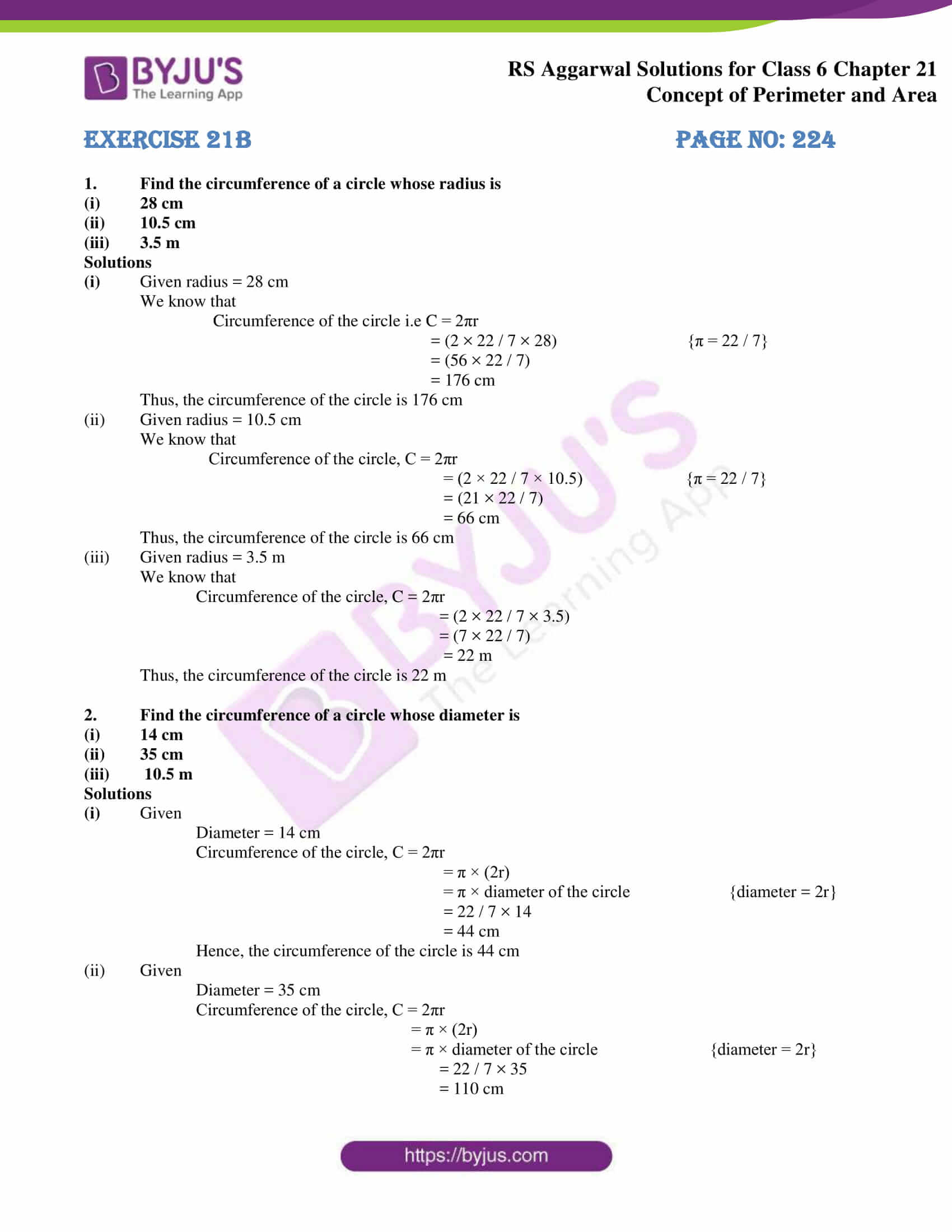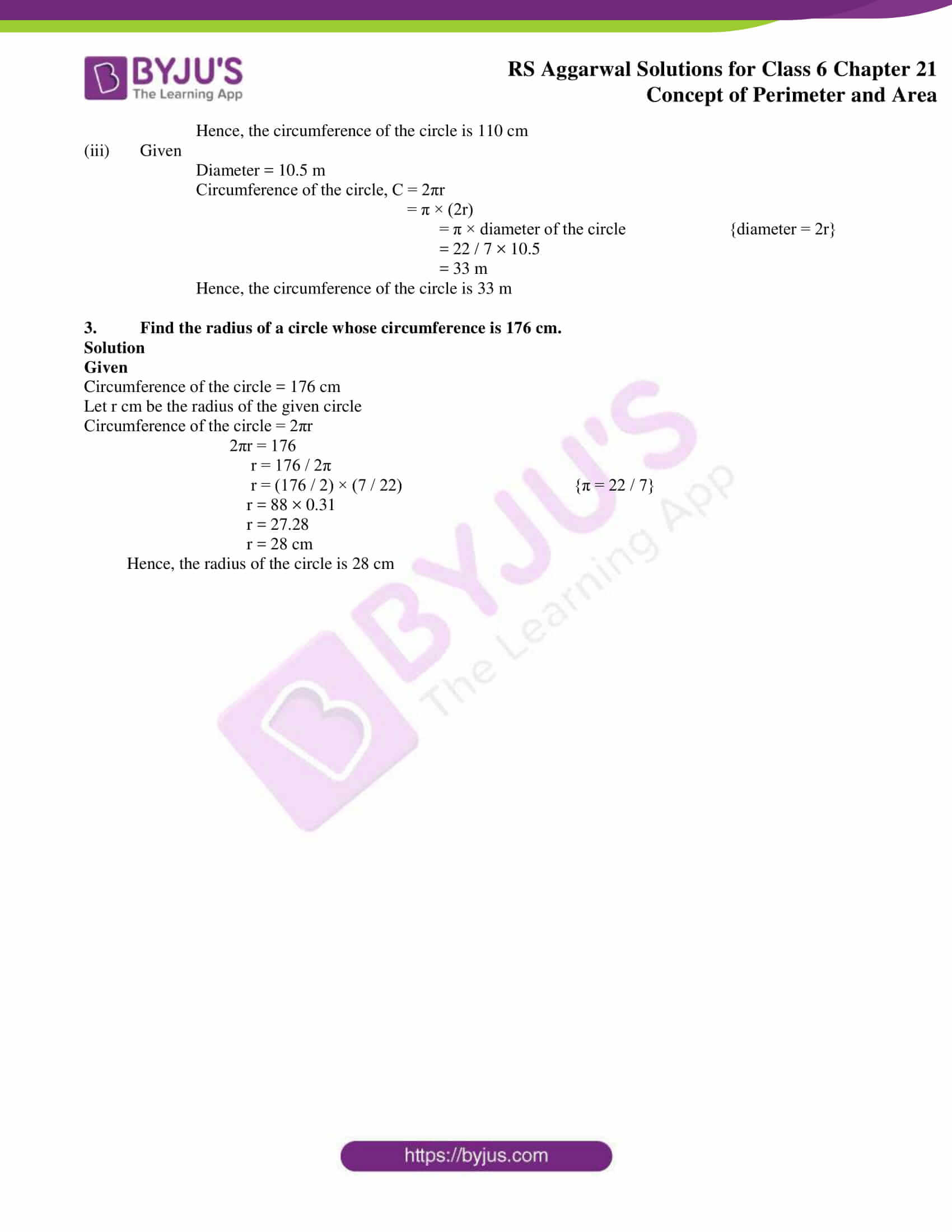# RS Aggarwal Solutions for Class 6 Chapter 21 Concept of Perimeter and Area Exercise 21B

We at BYJU’S strive hard intending to provide a better learning experience for the students. RS Aggarwal Solutions is a vital study material for the students, which is important from the exam point of view. This exercise mainly helps us to understand the steps which are followed in determining the circumference of a circle. For further reference, RS Aggarwal Solutions for Class 6 Chapter 21 Concept of Perimeter and Area Exercise 21B PDF can be downloaded here.

## Download PDF of RS Aggarwal Solutions for Class 6 Chapter 21 Concept of Perimeter and Area Exercise 21B### Access answers to Maths RS Aggarwal Solutions for Class 6 Chapter 21 Concept of Perimeter and Area Exercise 21B

1. Find the circumference of a circle whose radius is

(i) 28 cm

(ii) 10.5 cm

(iii) 3.5 m

Solutions

(i) Given radius = 28 cm

We know that

Circumference of the circle i.e C = 2πr

= (2 × 22 / 7 × 28) {π = 22 / 7}

= (56 × 22 / 7)

= 176 cm

Thus, the circumference of the circle is 176 cm

(ii) Given radius = 10.5 cm

We know that

Circumference of the circle, C = 2πr

= (2 × 22 / 7 × 10.5) {π = 22 / 7}

= (21 × 22 / 7)

= 66 cm

Thus, the circumference of the circle is 66 cm

(iii) Given radius = 3.5 m

We know that

Circumference of the circle, C = 2πr

= (2 × 22 / 7 × 3.5)

= (7 × 22 / 7)

= 22 m

Thus, the circumference of the circle is 22 m

2. Find the circumference of a circle whose diameter is

(i) 14 cm

(ii) 35 cm

(iii) 10.5 m

Solutions

(i) Given

Diameter = 14 cm

Circumference of the circle, C = 2πr

= π × (2r)

= π × diameter of the circle {diameter = 2r}

= 22 / 7 × 14

= 44 cm

Hence, the circumference of the circle is 44 cm

(ii) Given

Diameter = 35 cm

Circumference of the circle, C = 2πr

= π × (2r)

= π × diameter of the circle {diameter = 2r}

= 22 / 7 × 35

= 110 cm

Hence, the circumference of the circle is 110 cm

(iii) Given

Diameter = 10.5 m

Circumference of the circle, C = 2πr

= π × (2r)

= π × diameter of the circle {diameter = 2r}

= 22 / 7 × 10.5

= 33 m

Hence, the circumference of the circle is 33 m

3. Find the radius of a circle whose circumference is 176 cm.

Solution

Given

Circumference of the circle = 176 cm

Let r cm be the radius of the given circle

Circumference of the circle = 2πr

2πr = 176

r = 176 / 2π

r = (176 / 2) × (7 / 22) {π = 22 / 7}

r = 88 × 0.31

r = 27.28

r = 28 cm

Hence, the radius of the circle is 28 cm

### Access other exercises of RS Aggarwal Solutions for Class 6 Chapter 21 Concept of Perimeter and Area

Exercise 21E Solutions